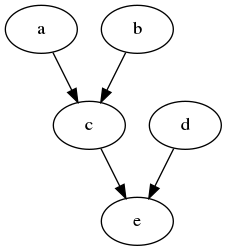# Algorithm

Structure learning of causal Bayesian Belief Networks (BBNs) using regression and sequence information has been reported [Ale20a, Ale20b]. In this section, we will take a less formal approach to explaining the structure learning algorithm. The structure learning algorithm is best understood when a complete ordering of a set of variables is given. Assume we have a causal model for which we know the true structure and parameters, and let’s say this model is a causal Bayesian Belief Network (BBN). Let’s say this model is shown in the figure below with the variables as all binary.Now, let’s say we have observed data from this causal BBN, and a sample of the data looks as below.

 1a,b,c,d,e
21,0,0,0,0
31,0,0,0,0
41,0,0,1,1
50,0,0,0,1
60,0,0,0,0
71,0,0,0,1
81,0,0,0,0
91,0,0,1,1
100,0,0,0,1
111,0,0,0,0


If a user can correctly specify the order of the variables by indicating which variable occurs before which other ones, then we can induce/learn a causal BBN structure from the data. Let’s say a user specifies the order to be a, b, c, d, e. Note that even though a does not come before b and vice-versa, that is okay, since they are tied and we just need an ordering.

The structure learning algorithm iterates over each variable as a dependent variable while regressing on all those that come before it. Since there are 5 variables, there are a maximum of 5 regression equations to run. Since a is the first variable and no other variables precede it, we will only run 4 regression equations.

• $$e = a + b + c + d$$

• $$d = a + b + c$$

• $$c = a + b$$

• $$b = a$$

We will eliminate which independents variables are not a parent of the dependent variable by knowing that the sequence implies time dependency and the coefficient associated with each independent variable indicates prediction strength. Lasso regularization will force the coefficients to zero, and it is expected that each model specified will have non-zero coefficients for those independent variables that are parents of the dependent variable. The following table lists the coefficients of each variable in a model for when the specified variable is the dependent variable.

Regression Parameters

Dependent Variable

a

b

c

d

e

a

0

0

0

0

0

b

0

0

0

0

0

c

0.8

0.3

0

0

d

0

0

0

0

0

e

0

0

0.3

0.8

0

You see that the regression models for a, b and d have no parents. The regression model for c as the dependent variable suggests that a and b are its parents. The regression model for e as the dependent variable suggests that c and d are its parents.

With the sequence to help us build the models, and with using Lasso regularization, we now can induce parent-child relationships between the dependent and non-zero coefficient variables (by non-zero, we mean the absolute value of the coefficient). We can proceed through the models from e to a (as the dependent variable) and start drawing the arcs between parent and child one at a time, and where a cycle is formed, skip drawing this arc.